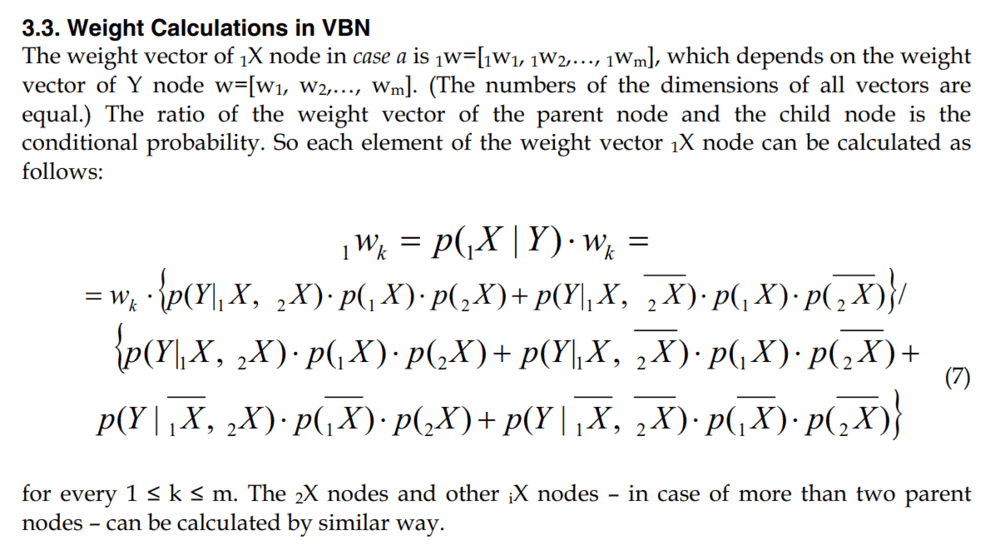# Can this be rearranged to solve?

• A
jeff91
Is it possible to rearrange or inverse this to solve for p(1X | Y)? I haven't done this level of maths for over 15 years and haven't a clue where to start.

1wk =p(1X | Y)⋅ wk

weight vector of 1X node in case a is 1w=[1w1, 1w2,…, 1wm]
weight vector of Y node w=[w1, w2,…, wm]

where 1 ≤ k ≤ m

I don't understand the notation. Are you asking about solving an equation of the form:

<n-dimensional column vector> = <n by n matrix> < another n-dimensional column vector>

You want to solve for the matrix when given the two column vectors?

•jeff91
Mentor
I don't understand the notation either.
If p(1X | Y) is a scalar then it's trivial to solve (you have m equations that should all lead to the same scalar, excluding possible lines that are 0=p*0). If it is an mxm matrix then there is not enough information to determine it uniquely.

•jeff91
Mentor
What is the context of your problem? It looks like something related to a neural net where the p(X|Y) is some kind of Baysian probability

•jeff91
jeff91
Thank you for your replies. I am trying to obtain probabilities to put in a Bayesian network from an AHP questionnaire.
This is the bit from the book I am looking at.I have the weights as they are outputted from the AHP spreadsheet.
I have only ever done Bayesian networks when I have been supplied with the probabilities.

Mentor
It's an mxm matrix then. No, you can't invert that problem. There are many different matrices that will lead to the same result for given vectors 1wk and wk.

•jeff91